`Used together with:`
calculus (22)
logic (15)
calculi (14)
cut (9)
proof (7)

## Stem sequent\$ (all stems)

### 43 papers: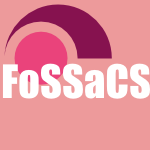FoSSaCS-2015-CloustonG #calculus
Sequent Calculus in the Topos of Trees (RC, RG), pp. 133–147.TLCA-2015-Santo #calculus #exclamation
Curry-Howard for Sequent Calculus at Last! (JES), pp. 165–179.POPL-2014-HouCGT #logic #proving
Proof search for propositional abstract separation logics via labelled sequents (ZH, RC, RG, AT), pp. 465–476.IJCAR-2014-LahavZ #calculus #satisfiability
SAT-Based Decision Procedure for Analytic Pure Sequent Calculi (OL, YZ), pp. 76–90.IJCAR-2014-Lindblad #calculus #higher-order #logic
A Focused Sequent Calculus for Higher-Order Logic (FL), pp. 61–75.IJCAR-2014-OlivettiP #calculus #implementation #logic #named
NESCOND: An Implementation of Nested Sequent Calculi for Conditional Logics (NO, GLP), pp. 511–518.FoSSaCS-2013-Strassburger #logic
Cut Elimination in Nested Sequents for Intuitionistic Modal Logics (LS), pp. 209–224.CSL-2013-CloustonDGT #calculus #linear #logic
Annotation-Free Sequent Calculi for Full Intuitionistic Linear Logic (RC, JED, RG, AT), pp. 197–214.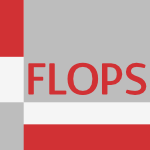FLOPS-2012-AriolaDHNS #calculus #call-by #semantics
Classical Call-by-Need Sequent Calculi: The Unity of Semantic Artifacts (ZMA, PD, HH, KN, AS), pp. 32–46.CSL-2012-ChaudhuriHM #approach #calculus
A Systematic Approach to Canonicity in the Classical Sequent Calculus (KC, SH, DM), pp. 183–197.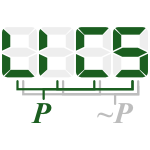LICS-2012-AvronKZ #calculus #composition #logic
Modular Construction of Cut-free Sequent Calculi for Paraconsistent Logics (AA, BK, AZ), pp. 85–94.LICS-2012-GargGN #calculus #logic #multi
Countermodels from Sequent Calculi in Multi-Modal Logics (DG, VG, SN), pp. 315–324.SAT-2012-Egly #on the
On Sequent Systems and Resolution for QBFs (UE), pp. 100–113.CSL-2010-Hetzl #calculus #representation
A Sequent Calculus with Implicit Term Representation (SH), pp. 351–365.IJCAR-2010-BrilloutKRW #calculus #quantifier
An Interpolating Sequent Calculus for Quantifier-Free Presburger Arithmetic (AB, DK, PR, TW), pp. 384–399.CSL-2009-Tatsuta #calculus #commutative #first-order
Non-Commutative First-Order Sequent Calculus (MT), pp. 470–484.LICS-2009-LiangM #calculus #proving
A Unified Sequent Calculus for Focused Proofs (CL, DM), pp. 355–364.CSL-2007-GaintzarainHLNO #calculus #invariant
A Cut-Free and Invariant-Free Sequent Calculus for PLTL (JG, MH, PL, MN, FO), pp. 481–495.LICS-2007-BrotherstonS #calculus #induction #infinity
Complete Sequent Calculi for Induction and Infinite Descent (JB, AS), pp. 51–62.TAP-2007-RummerS #calculus #java #logic #proving #source code #using
Proving Programs Incorrect Using a Sequent Calculus for Java Dynamic Logic (PR, MAS), pp. 41–60.TLCA-2007-SantoMP #calculus #continuation #normalisation
Continuation-Passing Style and Strong Normalisation for Intuitionistic Sequent Calculi (JES, RM, LP), pp. 133–147.ESOP-2006-SummersB #calculus #morphism #polymorphism
Approaches to Polymorphism in Classical Sequent Calculus (AJS, SvB), pp. 84–99.CSL-2006-LengrandDM #calculus #type system
A Sequent Calculus for Type Theory (SL, RD, JM), pp. 441–455.IJCAR-2006-DyckhoffKL #bound #calculus #logic
Strong Cut-Elimination Systems for Hudelmaier’s Depth-Bounded Sequent Calculus for Implicational Logic (RD, DK, SL), pp. 347–361.TLCA-2005-Hermant #calculus #semantics
Semantic Cut Elimination in the Intuitionistic Sequent Calculus (OH), pp. 221–233.LICS-2004-GabbayC #calculus #logic
A Sequent Calculus for Nominal Logic (MG, JC), pp. 139–148.ICALP-2003-GutierrezR #calculus #type system
Expansion Postponement via Cut Elimination in Sequent Calculi for Pure Type Systems (FG, BCR), pp. 956–968.TLCA-2003-SantoP #calculus #multi
Permutative Conversions in Intuitionistic Multiary Sequent Calculi with Cuts (JES, LP), pp. 286–300.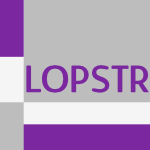LOPSTR-2002-GutierrezR #calculus #type system #verification
A Cut-Free Sequent Calculus for Pure Type Systems Verifying the Structural Rules of Gentzen/Kleene (FG, BCR), pp. 17–31.IJCAR-2001-BeckertS #calculus #first-order #logic
A Sequent Calculus for First-Order Dynamic Logic with Trace Modalities (BB, SS), pp. 626–641.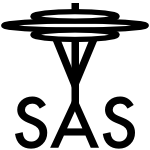SAS-2000-AmatoL #abstract interpretation #calculus #semantics
Abstract Interpretation Based Semantics of Sequent Calculi (GA, GL), pp. 38–57.CL-2000-Pliuskevicius #deduction #on the #strict
On an ω-Decidable Deductive Procedure for Non-Horn Sequents of a Restricted FTL (RP), pp. 523–537.CSL-2000-CoquandZ
Sequents, Frames, and Completeness (TC, GQZ), pp. 277–291.LICS-2000-Voronkov #calculus #how #logic #proving
How to Optimize Proof-Search in Modal Logics: A New Way of Proving Redundancy Criteria for Sequent Calculi (AV), pp. 401–412.WRLA-1998-Viry #calculus #equation
Adventures in sequent calculus modulo equations (PV), pp. 21–32.CSL-1997-BonattiO #calculus
A Sequent Calculus for Circumscription (PAB, NO), pp. 98–114.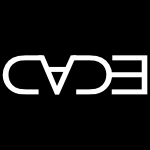CADE-1996-SchmittK #matrix #proving
Converting Non-Classical Matrix Proofs into Sequent-Style Systems (SS, CK), pp. 418–432.SEKE-1994-Sakalauskaite #branch #calculus #first-order #logic
A complete sequent calculus for a first order branching temporal logic (JS), pp. 274–280.CADE-1994-RichardsKSW #logic #named
Mollusc: A General Proof-Development Shell for Sequent-Based Logics (BLR, IK, AS, GAW), pp. 826–830.LICS-1994-LincolnS #calculus #first-order #linear #logic #proving
Proof Search in First-Order Linear Logic and Other Cut-Free Sequent Calculi (PL, NS), pp. 282–291.CADE-1992-Shankar #calculus #proving
Proof Search in the Intuitionistic Sequent Calculus (NS), pp. 522–536.JICSLP-1992-Cerrito #calculus #ll #unification
Herbrand Methods in Sequent Calculi: Unification in LL (SC), pp. 607–621.LICS-1990-LassezM #calculus #constraints
A Constraint Sequent Calculus (JLL, KM), pp. 52–61.

Bibliography of Software Language Engineering in Generated Hypertext (BibSLEIGH) is created and maintained by Dr. Vadim Zaytsev.
Hosted as a part of SLEBOK on GitHub.